### 13.4.1.4 Procedure for deriving the state transition equation

The following general procedure can be followed to derive the differential model using Lagrangian mechanics on a coordinate neighborhood of a smooth-dimensional manifold:

1. Determine the degrees of freedom of the system and define the appropriate-dimensional smooth manifold.
2. Express the kinetic energy as a quadratic form in the configuration velocity components: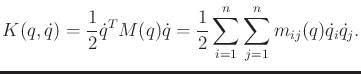(13.137)

3. Express the potential energy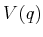.
4. Let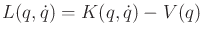be the Lagrangian function, and use the Euler-Lagrange equation (13.130) to determine the differential constraints.
5. Convert to phase space form by letting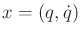. If possible, solve for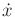to obtain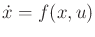.

Example 13..12 (2D Rigid Body Revisited)

The equations in (13.109) can be alternatively derived using the Euler-Lagrange equation. Let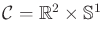, and let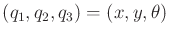to conform to the notation used to express the Lagrangian.

The kinetic energy is the sum of kinetic energies due to linear and angular velocities, respectively. This yields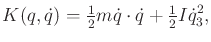(13.138)

in which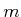and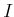are the mass and moment of inertia, respectively. Assume there is no gravity; hence,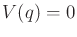and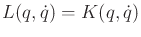.

Suppose that generalized forces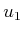,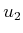, and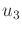can be applied to the configuration variables. Applying the Euler-Lagrange equation to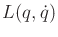yields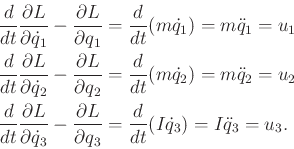(13.139)

These expressions are equivalent to those given in (13.109). One difference is that conversion to the phase space is needed. The second difference is that the action variables in (13.139) do not refer directly to forces and moments. They are instead interpreted as generalized forces that act on the configuration variables. A conversion should be performed if the original actions in (13.109) are required.Steven M LaValle 2020-08-14